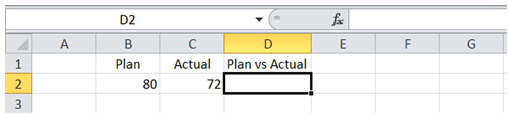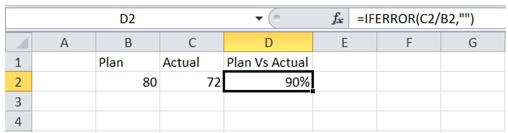## Vba add relative formula in cell in Excel

For example, I want to enter a formula "=IFERROR(C2/B2,"") in cell D2 as shown below.To do it in Excel, here is the answer:

1. Option Explicit
3. ActiveSheet.Range("D2").Formula = "=Iferror(RC[-1]/RC[-2],"""")"
4. End Sub

Description:

a) Line 3 adds the formula in Range D2.

b) C2 is one Column to the left of D2 in the same row. So, it is entered as RC[-1].

c) B2 is two Columns to the left of D2 in the same row. So, it is entered as RC[-2].

d) Notice the use of """" to introduce a quote within the formula string.

Result after Macro execution: